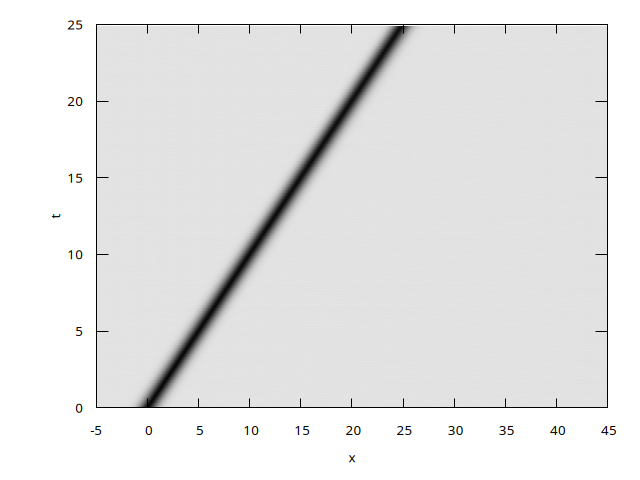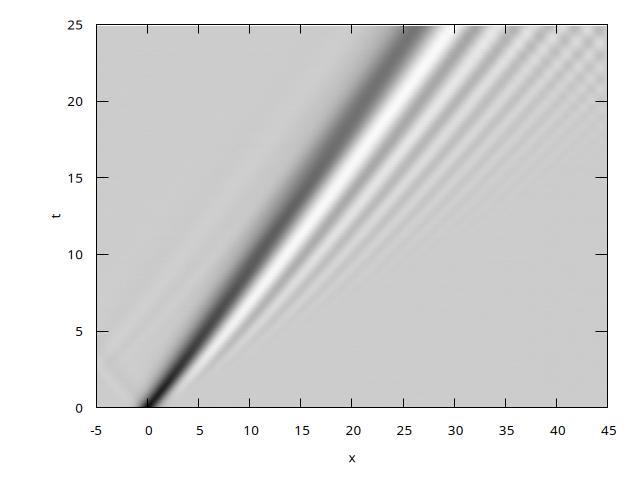# Wave equation with dispersion due to capillarity

Explicit resolution of linearized Saint Venant with dispersion \displaystyle \frac{\partial h}{\partial t}+ \frac{\partial hu}{\partial x}=0 \displaystyle \frac{\partial hu}{\partial t}= - \frac{\partial h}{\partial x} + \sigma \frac{\partial^3 h}{\partial x^3} Note that there are no nonlinear terms \frac{\partial hu^2}{\partial x} in the total derivative d_t hu of momentum for the moment (hu is the flux, h is the height of water, \sigma the surface tension parameter).

//#include "grid/cartesian1D.h"
#include "grid/multigrid1D.h"
#include "run.h"

scalar h[];
face vector hu[];
double dt, sigma;
FILE * out;

Outflow boundary conditions.

hu.n[left] = neumann(0);
h[left] = neumann(0);
hu.n[right] = neumann(0);
h[right] = neumann(0);

Parameters, siez of domain, origin, number of points, time step

int main() {
L0 = 50.;
X0 = -5;
N = 512;
DT = 5e-5;

Loop on surface tension \sigma. Results are written in a file indexed by surface tension.

    for (sigma = 0.; sigma <= 0.1; sigma += 0.1) {
char name;
sprintf (name, "out-%g", sigma);
out = fopen (name, "w");
run();
}
}

The initial field is a linear wave moving to the right.

event init (t = 0) {
#define EPS 0.01
foreach()
h[] = 1. + EPS*exp(-x*x);
foreach_face()
hu.x[] = EPS*exp(-x*x);
boundary ({h,hu});
}

Output fields for plots.

event printdata (t += .1; t <= 25) {
foreach()
fprintf (out, "%g %g %g %g\n", x, h[], hu.x[], t);
fprintf (out, "\n");
}

Integration.

event integration (i++) {
double dt = DT;
scalar u[];
foreach_face() {
u[] = 2.*hu.x[]/(h[] + h[-1,0]);
double un = fabs(u[]);
if (un > 0. && CFL*Delta/un < dt)
dt = CFL*Delta/un;
}
dt = dtnext ( dt);

Compute the curvature K = \frac{\partial^2 h}{\partial x^2}

    scalar K[];
foreach()
K[] = (h[1,0] + h[-1,0] - 2.*h[])/sq(Delta);
boundary ({K});

Curvature contribution to pressure and hydro \displaystyle \frac{\partial hu}{\partial t}= - \frac{\partial h}{\partial x} + \sigma \frac{\partial K}{\partial x}

    foreach_face()
hu.x[] += dt*(- (h[] - h[-1,0]) + sigma*(K[] - K[-1,0]))/Delta;

Variation of h \displaystyle \frac{\partial h}{\partial t}+ \frac{\partial hu}{\partial x}=0

    scalar dh = K; // we just reuse K as dh
foreach()
dh[] = ((u[] < 0. ? h[] : h[-1,0])*u[] -
(u[1,0] > 0. ? h[] : h[1,0])*u[1,0])/Delta;
foreach()
h[] += dt*dh[];
boundary ({h,hu});
}

# Run

 qcc -g -O3 -o disperse disperse.c -lm
./disperse

or with make

 make disperse.tst;make disperse/plots
make disperse.c.html ; open disperse.c.html 

# Results

The abscissa is x the ordonate is time t.

First we verify the propagation of a pulse which does not change during the propagation

 set output 'dalembert.png'
set pm3d map
set palette gray negative
unset colorbox
set tmargin at screen 0.95
set bmargin at screen 0.15
set rmargin at screen 0.95
set lmargin at screen 0.15
set xlabel "x"
set ylabel "t"
unset key
splot 'out-0' u 1:4:2The d’Alembert solution, (script)

Second we observe that dispersion distroys the signal and breaks it in small waves traveling faster. Note the initial wave generates a small one running left and reflecting on the border.

 set output 'disperse.png'
set pm3d map
set palette gray negative
unset colorbox
set tmargin at screen 0.95
set bmargin at screen 0.15
set rmargin at screen 0.95
set lmargin at screen 0.15
set xlabel "x"
set ylabel "t"
unset key
splot 'out-0.1' u 1:4:2Propagation with dispersion (script)

# Next steps

• couple with Shallow Water solvers
• compare to the case with \sigma negative (see mascaret)

ready for new site 09/05/19